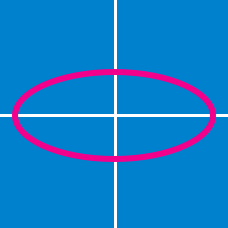Geometry

# Conics - Hyperbola

Consider a branch of the hyperbola $x^2-2y^2-2\sqrt{5}x-4\sqrt{2}y-3=0$ with vertex at a point $$A.$$ Also, let $$B$$ be one of the end points of its latus rectum. If $$C$$ is the focus of the hyperbola nearest to point $$A,$$ what is the area of triangle $$ABC ?$$

What are the coordinates of the foci of the rectangular hyperbola $x^2-y^2=7 ^2?$

What are the coordinates of the vertices of the hyperbola $4x^2-9y^2-40x+54y -17=0 ?$

Given points $$F=(5,0)$$ and $$F'=(-5,0),$$ what is the equation of the locus of points $$P$$ in a plane such that $\left\lvert \left\lvert \overline{PF} \right\rvert - \left\lvert \overline{PF'} \right\rvert \right\rvert= 8 ?$

Note: $$\lvert \overline{AB} \rvert$$ denotes the length of line segment $$\overline{AB}.$$

Suppose that $$(7,12)$$ and $$(11,8)$$ are the foci of a hyperbola passing through the origin. Then the eccentricity of the hyperbola is $$\text{_________}.$$

×

Problem Loading...

Note Loading...

Set Loading...